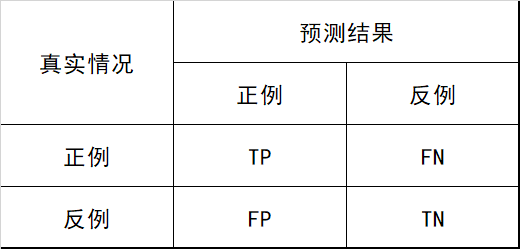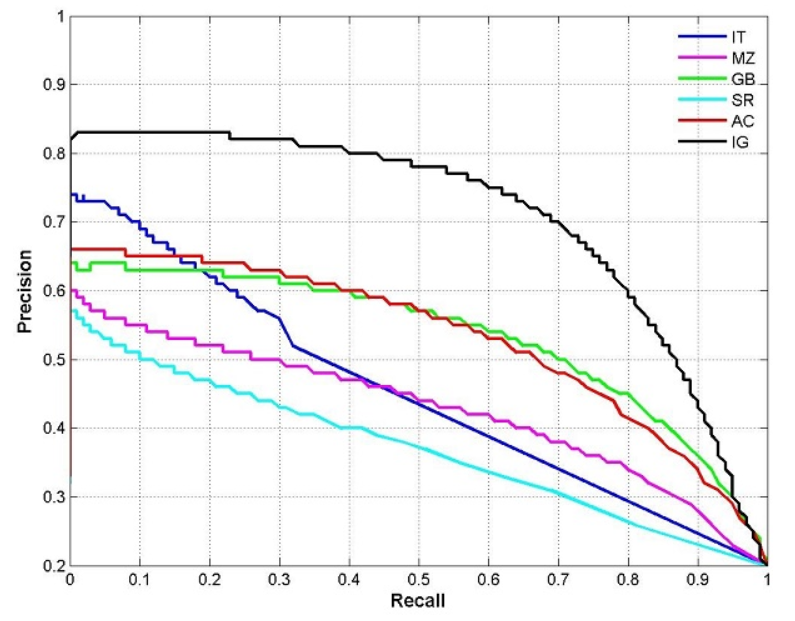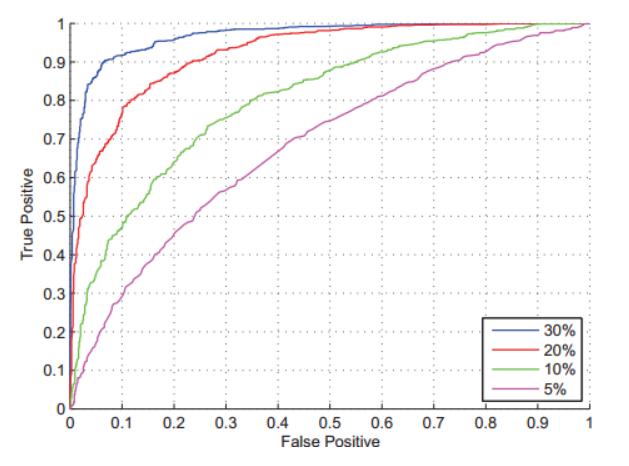# 评估指标¶

## 基础¶## 精度¶

$Accuracy=\frac{TP+TN}{TP+FN+FP+TN}$

## 精确率¶

$Precision=\frac{TP}{TP+FP}$

## 召回率¶

$Recall=\frac{TP}{TP+FN}$

## P-R曲线¶

P-R曲线又叫做：PRC## F1 值¶

$F1=\frac{2 * P * R}{P + R}$

## TPR¶

$TPR=\frac{TP}{TP+FN}$

## FPR¶

$FPR=\frac{FP}{TN+FP}$

## AUC¶

Area Under ROC Curve，ROC曲线下的面积：## 敏感性¶

$sensitivity =\frac{TP}{TP + FN}$

## 特异性¶

$specificity =\frac{TN}{TN + FP}$